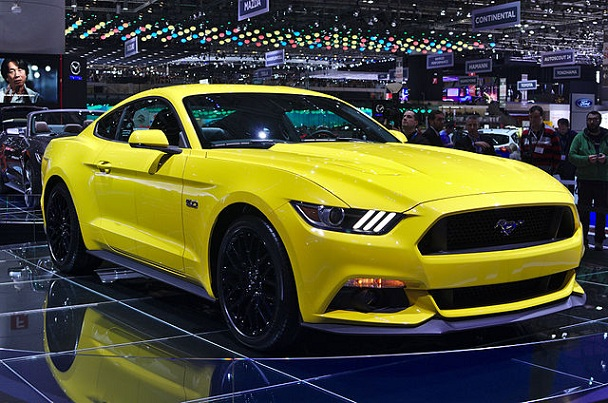# Difference Between Horsepower and Torque

## Main Difference – Horsepower vs. Torque

Horsepower and torque are two specifications that are often used to classify vehicles. The main difference between horsepower and torque is that horsepower measures how much energy an engine produces per unit time, whereas torque measures the twisting force that the engine creates.Horsepower and torque are used to give specifications to cars. For instance, the 2015 Ford Mustang, shown above, has a horsepower of 435 hp at 6500 rpm and a torque of 320 lb.-ft. at 3000 rpm.

## What is Horsepower

Horsepower is a unit for measuring power, i.e. how much energy is produced by the engine per unit time. It is not the SI unit for measuring power: the SI unit is the watt. Incidentally, it was James Watt, after whom the SI unit is named after, that came up with the concept for the unit of horsepower. Under the definition for horsepower, if a horse is able to do work at a rate of 33000 foot-pounds per minute then the horse has a power of 1 horsepower (1 hp). A foot-pound is a unit for measuring work done. Simply, it is an amount of force (measured here in pounds) multiplied by an amount of distance (measured here in feet).

## What is Torque

Torque is a measurement of twisting force that an engine can produce. It is given in units of foot-pounds. Since it is the twisting force that eventually gets transmitted to the wheels and gets the vehicle moving, torque tells us about how easy it is to get a vehicle to move or for it to change its speed.

## Relationship Between Torque and Horsepower

Horsepower and torque are related:$\mathrm{Horsepower=\frac{Torque\:\times \:RPM}{5252}}$

Here, RPM is the number of complete revolutions that the crankshaft of an engine rotates through per minute.

As the RPM of an engine increases from a low value, the torque also increases, and eventually takes on a more or less steady value. If the RPM is increased further beyond this steady stage the torque decreases once again. Even while the torque is steady, the horsepower continues to increase for a larger range of RPMs as it is proportional to the product of RPM and torque.

Usually, how the torque and horsepower vary with RPM is largely dependent on the engine design. Some vehicles are designed to maintain their peak torque over a larger range of RPMs. The graph below depicts how torque and horsepower change as the RPM changes, for two different types of engines:Graphs of horsepower and torque vs. RPM

Note that torque is equal to horsepower when RPM ≈5252.

## Difference Between Horsepower and Torque

### What it Measures

Horsepower measures the engine’s power.

Torque measures the twisting force produced by the engine.

### Units

Horsepower itself is a unit. Foot-pounds per minute are equivalent units for horsepower.

Torque is a quantity, and it is measured in foot-pounds.

Image Courtesy

“Salon de l’auto de Genève 2014 – 20140305 – Ford” by Clément Bucco-Lechat (Own work) [CC BY-SA 3.0], via Wikimedia Commons

“File:Powerband.gif”, author unknown [CC BY-SA 3.0], via Wikimedia Commons